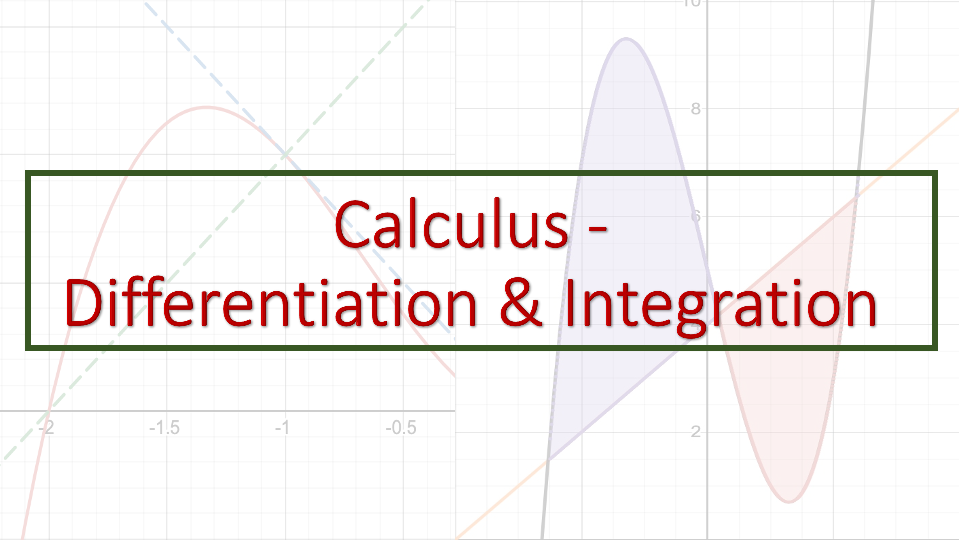## Calculus - Differentiation & Integration

This course is designed for students looking to learn Differentiation & Integration. The course has the following learning materials.
• Course content video explaining the concept
• Course content notes summarizing the video
• End of Section Worksheet and its Solution
• Worksheet solution explanation video

#### What you’ll learn?

• Limits
• Differentiation of a Function
• Derivative Rules
• Tangents and Normal
• Graphical Interpretation
• Application of Derivatives
• Logarithms & Exponents
• AntiDifferentiation
• Definite Integrals
• Integration by Substitution
• Integration by Parts• Curriculum
IB, A-Level, AP
• Level